Science
Technology |

# 10 Fun Examples of Recreational Number Theory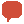. . . Comments

Mathematicians like to classify and organize numbers in all kinds of ways. Natural numbers are used for counting and ordering; nominal numbers are used for naming (like a driver’s license number); integers are numbers that can be expressed without a fraction or decimal; prime numbers can only divided by 1 and by themselves; and so on. But there is no limit to how we can understand and use numbers; accordingly, there is a branch of pure mathematics, primarily based upon the study of integers, called “number theory.” Though we now understand that number theory has boundless applications, uses, and purposes, it can appear to be frivolous to the point of pointlessness – especially the subset known as “recreational number theory.” Number theorist Leonard Dickson once said, after all, “Thank God that number theory is unsullied by any application.”

But that doesn’t mean it doesn’t provide a measure of nerdy fun for those so inclined. Read on to learn what makes a number “interesting,” “weird,” “happy,” “narcissistic,” “perfect,” and more!

10

Amicable numbers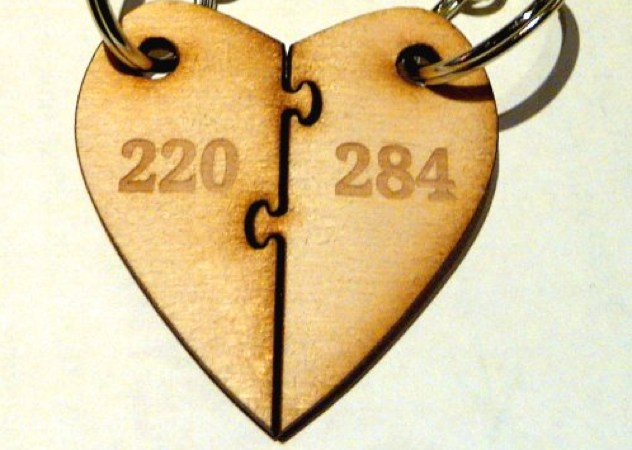Ah, amicable numbers. They love each other so much. How much? Well, let’s take a classic pair—284 and 220—and see just how friendly they are. Let’s take all the proper divisors of 220 (that is to say, all its divisors that leave no remainder, including the number 1, and excluding the number itself) and all them up:

1 + 2 + 4 + 5 + 10 + 11 + 20 + 22 + 44 + 55 + 110 = 284

Now, let’s take 284 and do the same thing:

1 + 2 + 4 +71 + 142 = 220.

Voila: a pair of amicable numbers. Other pairs include (1184, 1210), (2620, 2924), and (5020, 5564). This type of number pair was discovered and studied by the Pythagoreans, and has been the subject of much research through the centuries – Fermat, Descartes, Iranian Muhammad Baqir Yazdi, and Iraqi Th?bit ibn Qurra are among the many mathematicians who have delved into the world of amicable numbers. Topics of further study include attempts to discover if there is an infinite amount of pairs, to discern patterns, and to better understand why and how this happens.

Because mathematicians would never be satisfied with mere amicable numbers, “betrothed numbers” are pairs where the sum of the proper divisors of each number is equal to the other number +1.

9

EmirpEmirp” is the word “prime” spelled backwards, and it refers to a prime number that becomes a new prime number when you reverse its digits. Emirps do not include palindromic primes (like 151 or 787), nor 1-digit primes like 7. The first few emirps are 13, 17, 31, 37, 71, 73, 79, 97, 107, 113, 149, and 157 – reverse them and you’ve got a new prime number on your hands.

Mostly, saying “emirp” over and over is kind of a blast. Give it a whirl!

8

Interesting numbers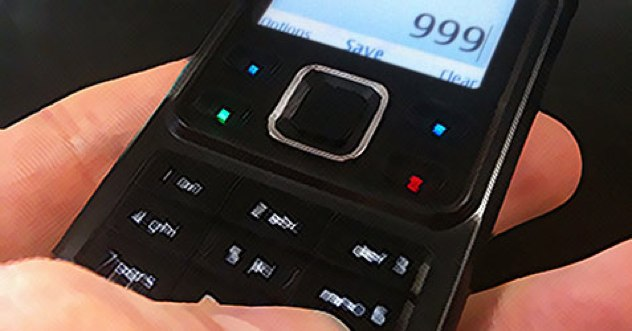There is an old paradox in the world of mathematics that is known as the “interesting number paradox.” Simply put, if you keep counting natural numbers, eventually you’ll encounter one that isn’t interesting; where it gets paradoxical is that by virtue of being the smallest uninteresting number, that number has now become interesting.

Of course, this is all subjective, as it relies on a vague definition of the word “interesting.” Very generally speaking, a number is considered interesting if it has some type of mathematical quality that sets it apart; 19 is interesting because it’s prime, 999 is interesting because it’s a palindrome (and the UK version of 911); 24 is interesting because (among other reasons) it’s the largest number divisible by all numbers less than its square root. Mathematicians

7

Powerful numbersAchilles was a powerful Trojan War hero who was extremely powerful but had one flaw—his achilles heel. Like him, Achilles numbers are powerful but not perfect.

So, let’s begin with a powerful number. A number is considered powerful if all of its prime factors remain factors once they are squared. 25 is a powerful number because its one prime factor, 5, remains a factor once its been squared (25, which goes into 25 once). Now let’s move onto perfect powers, number that can be expressed as an integer power of another integer; 8 is a perfect power, as it’s 2 cubed.

So now, back to the original premise – Achilles numbers are powerful, but they are not perfect powers. 72 is the first Achilles number, as it is powerful, but it is not a perfect prime. Others include 108, 200, 288, 392, 432, 500, and 648.

6

Weird numbers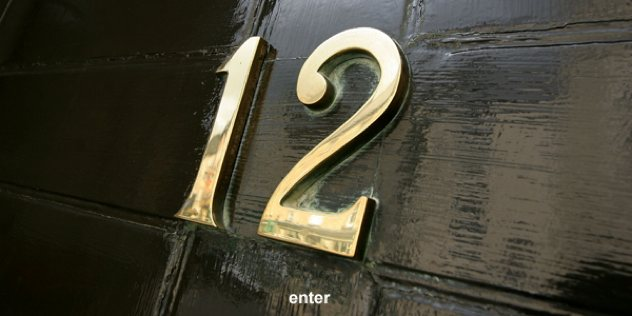What are weird numbers? To understand them, we must first begin with “abundant” numbers. Abundant numbers, also known as “excessive,” are bigger than the sum of their proper divisors. 12, for instance, is the first (smallest) abundant number—the sum of its proper divisors, 1+2+3+4+6, is 16. 12, therefore, has an “abundance” of 4, the amount by which the sum of its divisors exceeds the number. There are many even abundant numbers, but we don’t get to an odd one until the number 945.

Some abundant numbers are “semiperfect” or “pseudoperfect,” meaning that they are equal to all or just some of their proper divisors. 12 is an imperfect abundant number because some of its divisors can be added together to form 12.

At last, we arrive at weird numbers. A number is weird if it is abundant but NOT semiperfect; in other words, the sum of its divisors is larger than the number itself, but no subset of divisor sums equal the number. Weird numbers are uncommon – the first few are 70, 836, 4,030, and 5,830.

5

Untouchable numbers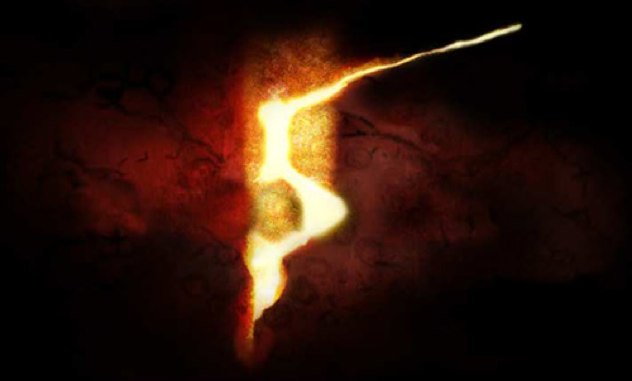While weird numbers are not equal to the sum of any of their divisors, untouchable numbers take it a step further. For a number to be untouchable, it must not be equal to the sum of the proper divisors of ANY number. A few untouchables are 2, 5, 52, and 88; in fact, 5 is thought to be the only odd untouchable number in existence (though it hasn’t been formally proven). There are an infinite number of untouchable numbers, meaning there is no such thing as the largest one.

4

Perfect Numbers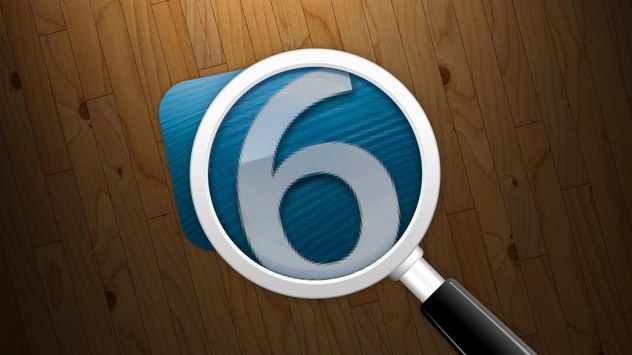So having discussed the weird and the untouchable, it’s time to check in with the grandaddy of all proper divisor-related numbers: perfect numbers. A perfect number is one that is exactly equal to the sum of its proper divisors (again, excluding itself). The first perfect number is 6, as its divisors (1, 2, 3) all up to 6. Six is followed by 28, 496, and 8,128. Early Greek mathematicians knew only of these first 4 perfect numbers; Nichomatus discovered 8,128 by the year A.D. 100. Three more were discovered, the first circa 1456 (33,550,336) by an unknown mathematician, and in 1588 (8,589,869,056 and 137,438,691,328) by Italian mathematician Pietro Cataldi in 1588.

All known perfect numbers are even; it is not yet known whether an odd prime exists or is even possible. English mathematician James Joseph Sylvester wrote “…a prolonged meditation on the subject has satisfied me that the existence of any one such [odd perfect number]—its escape, so to say, from the complex web of conditions which hem it in on all sides—would be little short of a miracle.”

3

Happy Numbers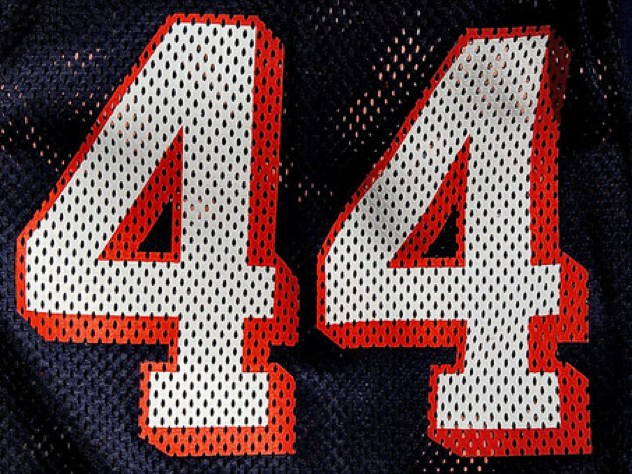Some numbers are weird; others are happy. If you’d like to find out if a given number is happy, you’ll need to perform the following set of operations. Let’s take the number 44:

First, square each digit, then add them together:

4^2 + 4^2 = 16 + 16 = 32

Then, we’ll do it again with our new number:

3^2 + 2^2 = 9 + 4 = 13

And again:

1^2 + 3^2 = 1 + 9 = 10

And finally:

1^2 + 0^2 = 1 + 0 = 1

Voila! It’s a happy number. Anytime you take a number, perform this “procedure,” and eventually arrive at the number 1, you have yourself a happy number. If your number never reaches 1, then sadly, it’s unhappy. Interestingly, happy number are extremely common; there are 11 of them between 1 and 50, for example.

As a final note, the greatest happy number with no recurring digits is 986,543,210. That is a happy number indeed.

2

Narcissistic numbers

Narcissistic numbers, also known as Armstrong numbers or “pluperfect digital invariants,” are numbers that—listen closely—are equal to the sum of each of its digits when those digits are raised to the power of the AMOUNT of digits in the number.

Ok. What? Let’s take an example of the four existing narcissistic cubes:

153 = 1^3 + 5^3 + 3^3
370 = 3^3 + 7^3 + 0^3
371 = 3^3 + 7^3 + 1^3
407 = 4^3 + 0^3 + 7^3

In these cases, each digit is cubed because there are three digits in the number. Then, those cubed numbers are added together to produce a sum equal to the original number. There are no 1-digit narcissistic numbers, nor 12 or 13-digit ones; the two 39-digit ones are:

115132219018763992565095597973971522400 and 115132219018763992565095597973971522401.

English mathematician G. H. Hardy recognized the frivolity of such numbers by proclaiming in his book “The Mathematician’s Apology” that “These are odd facts, very suitable for puzzle columns and likely to amuse amateurs, but there is nothing in them which appeals to the mathematician.”

1

Repdigits and repunits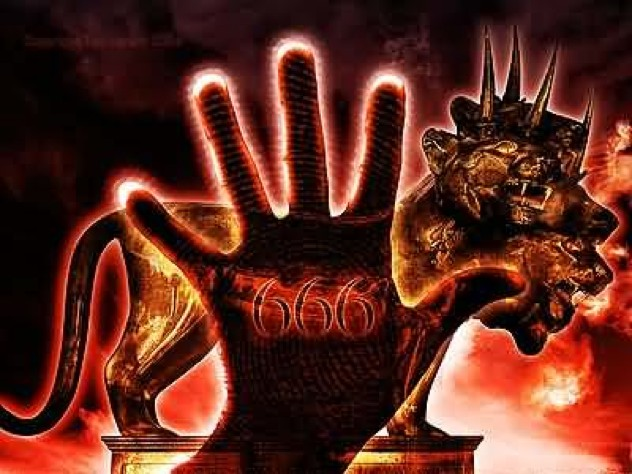A repdigit is a natural number with one repeating digit; the name, in fact, comes from the term “repeated digit.” The most famous redigit is the so-called “Beast Number” 666, a common symbol of the antichrist or of Satan. A repunit, then, is a repdigit that only uses the number 1; repunits pop up frequently in binary code and are related to that most famous of primes, Mersenne Primes. It has been conjectured that there are an infinite number of repunit primes, so if you’d like to try to prove it, please do so at your leisure.